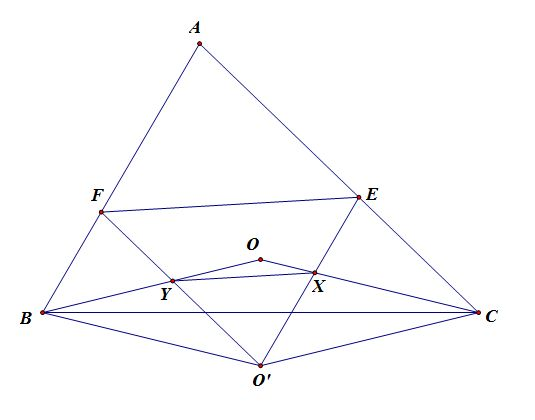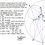# A cool property regarding circumcenter

Let $$O$$ be the circumcenter of $$ABC$$. Reflect $$O$$ over $$BC$$ to obtain $$O'$$. Through $$O'$$ construct lines parallel to $$AC,AB$$ which respectively meet $$AB,AC$$ at $$F,E$$. Define $$O'F\cap OB=Y, O'E\cap CO=X$$. Prove $$XY||EF$$I personally think this configuration is very rich and can be exploited to create difficult olympiad geo problems.Note by Xuming Liang
5 years, 8 months ago

This discussion board is a place to discuss our Daily Challenges and the math and science related to those challenges. Explanations are more than just a solution — they should explain the steps and thinking strategies that you used to obtain the solution. Comments should further the discussion of math and science.

When posting on Brilliant:

• Use the emojis to react to an explanation, whether you're congratulating a job well done , or just really confused .
• Ask specific questions about the challenge or the steps in somebody's explanation. Well-posed questions can add a lot to the discussion, but posting "I don't understand!" doesn't help anyone.
• Try to contribute something new to the discussion, whether it is an extension, generalization or other idea related to the challenge.
• Stay on topic — we're all here to learn more about math and science, not to hear about your favorite get-rich-quick scheme or current world events.

MarkdownAppears as
*italics* or _italics_ italics
**bold** or __bold__ bold
- bulleted- list
• bulleted
• list
1. numbered2. list
1. numbered
2. list
Note: you must add a full line of space before and after lists for them to show up correctly
paragraph 1paragraph 2

paragraph 1

paragraph 2

[example link](https://brilliant.org)example link
> This is a quote
This is a quote
    # I indented these lines
# 4 spaces, and now they show
# up as a code block.

print "hello world"
# I indented these lines
# 4 spaces, and now they show
# up as a code block.

print "hello world"
MathAppears as
Remember to wrap math in $$ ... $$ or $ ... $ to ensure proper formatting.
2 \times 3 $2 \times 3$
2^{34} $2^{34}$
a_{i-1} $a_{i-1}$
\frac{2}{3} $\frac{2}{3}$
\sqrt{2} $\sqrt{2}$
\sum_{i=1}^3 $\sum_{i=1}^3$
\sin \theta $\sin \theta$
\boxed{123} $\boxed{123}$

## Comments

Sort by:

Top Newest

Notice that $BOCO'$ is a parallelogram. Since $BP || O'C$ and $AB || O'E$, we see that $\angle FBX = \angle EO'C$. It is easy to see that $\angle A = \angle BFX = \angle YEC$. Therefore, $\triangle BFX \sim \triangle O'EC$. This implies that $\frac{BF}{FX} = \frac{O'E}{EC}$.

Similarly, we have that $\triangle YCE \sim \triangle BO'F \implies \frac{O'F}{FB} = \frac{CE}{EY}$.

Multiplying the two ratios completes the proof.

- 5 years, 8 months ago

Log in to reply

Yes! The parallel property will hold as long as $BOCO'$ is a parallelogram. There are a couple of typos in your proof, otherwise you got it spot on.

- 5 years, 8 months ago

Log in to reply

PS: The problem can be generalized.

- 5 years, 8 months ago

Log in to reply

Can you post the proof or hint after a few days?

- 5 years, 8 months ago

Log in to reply

Yes. :)

- 5 years, 8 months ago

Log in to reply

@Alan Yan Hints: We want to prove some two ratios are equal, perhaps look for some similar triangles from all the parallels.

Generalize this property if you get it.

- 5 years, 8 months ago

Log in to reply

×

Problem Loading...

Note Loading...

Set Loading...